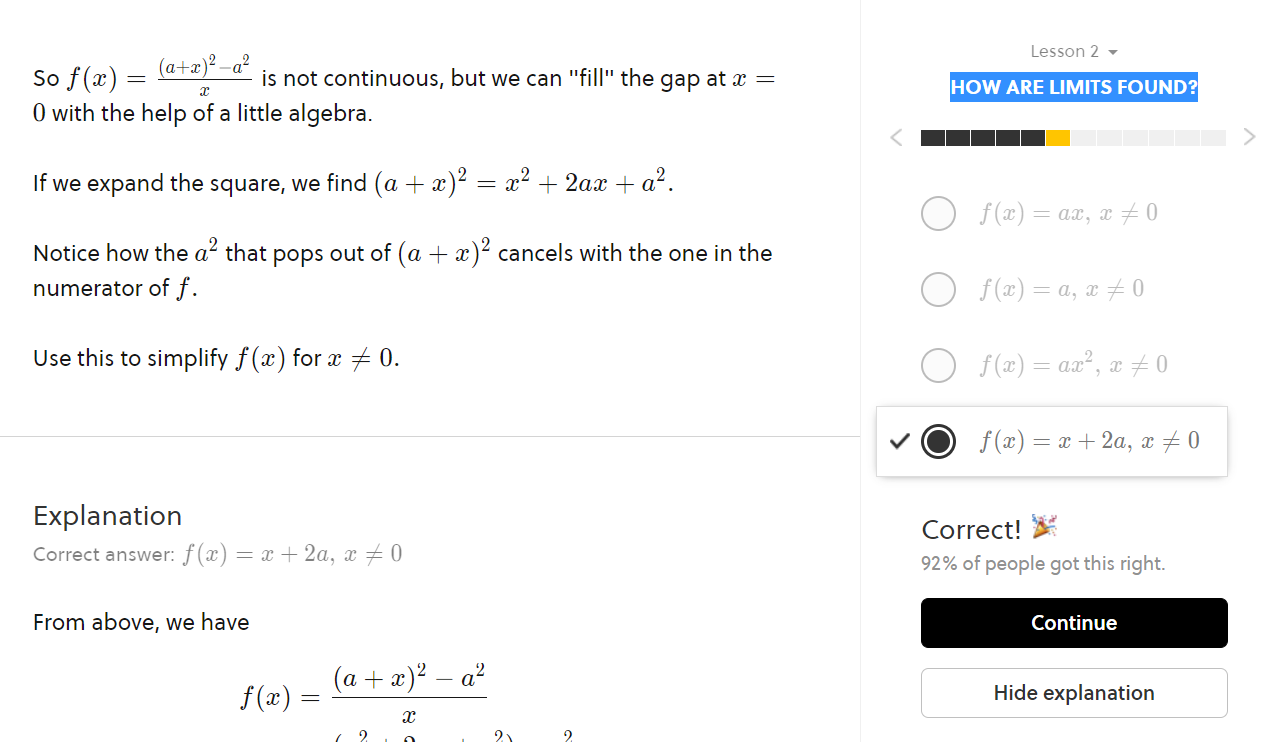# Discontinuity points. Who was born first? The egg or the chicken?

Hi I started doing the "Calculus in a Nutshell" because even if I passed the exam at university I still have a lot of doubts about my understanding. So when I answered this question I had a new doubt. As you can see the Function since has x at denominator has to have a point of discontinuity for x=0. But since this function is equivalent to a straight line if I only see the straight line function I should have no discontinuity points. So my question is... If someone shows me a function like the one in the answer and ask me if there are discontinuity points and I answer that there are none I am wrong because I can lead back the function to the one with a denominator? Or it's just a matter of who was born first? The egg or the chicken?

I apologize for my bad English.Note by - -
4 months, 1 week ago

This discussion board is a place to discuss our Daily Challenges and the math and science related to those challenges. Explanations are more than just a solution — they should explain the steps and thinking strategies that you used to obtain the solution. Comments should further the discussion of math and science.

When posting on Brilliant:

• Use the emojis to react to an explanation, whether you're congratulating a job well done , or just really confused .
• Ask specific questions about the challenge or the steps in somebody's explanation. Well-posed questions can add a lot to the discussion, but posting "I don't understand!" doesn't help anyone.
• Try to contribute something new to the discussion, whether it is an extension, generalization or other idea related to the challenge.

MarkdownAppears as
*italics* or _italics_ italics
**bold** or __bold__ bold
- bulleted- list
• bulleted
• list
1. numbered2. list
1. numbered
2. list
Note: you must add a full line of space before and after lists for them to show up correctly
paragraph 1paragraph 2

paragraph 1

paragraph 2

[example link](https://brilliant.org)example link
> This is a quote
This is a quote
    # I indented these lines
# 4 spaces, and now they show
# up as a code block.

print "hello world"
# I indented these lines
# 4 spaces, and now they show
# up as a code block.

print "hello world"
MathAppears as
Remember to wrap math in $$ ... $$ or $ ... $ to ensure proper formatting.
2 \times 3 $2 \times 3$
2^{34} $2^{34}$
a_{i-1} $a_{i-1}$
\frac{2}{3} $\frac{2}{3}$
\sqrt{2} $\sqrt{2}$
\sum_{i=1}^3 $\sum_{i=1}^3$
\sin \theta $\sin \theta$
\boxed{123} $\boxed{123}$

Sort by:

Is it possible to give down the function you had difficulties with? (You haven’t given the function in the note)

- 4 months, 1 week ago

I posted a screenshot in the post but I'll write it down anyway the function is $f(x)=\frac{(x+a)^2-a^2}{x}$ can be rewritten as $f(x)=x+2a$ with $x\neq0$

- 4 months, 1 week ago

- 4 months, 1 week ago

If someone were to show you the function $f(x) = x + 2a$, you would certainly be correct in saying that there are no discontinuity points. However, if someone were to show you the function $f(x) = x + 2a, ~\boldsymbol{x \neq 0}$, then you would not be able to give a correct answer. Whoever was showing you this function would be guilty of not giving you enough information. In other words, they would be asking you a question about all points on $f(x)$, but not telling you what happens when $x = 0$. So it would be impossible to give an answer. You could perhaps guess that there was a discontinuity point, since they told you that $f(x)$ was only correct when $x \neq 0$, which is "suspicious". But the only way to indicate that there is a discontinuity at $x = 0$ is to give the full function, $f(x) = \frac{(a+x)^2 - a^2}{x}$ . Hope that helps!

- 4 months, 1 week ago

Yup it helped. Thank you very very much!

- 4 months, 1 week ago

No problem!

- 4 months, 1 week ago